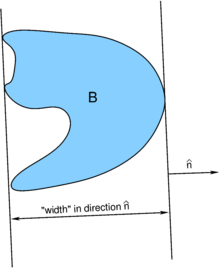# Mean width

In geometry, the mean width is a measure of the "size" of a body; see Hadwiger's theorem for more about the available measures of bodies. Indimensions, one has to consider-dimensional hyperplanes perpendicular to a given directionin, whereis the n-sphere (the surface of a-dimensional sphere). The "width" of a body in a given directionis the distance between the closest pair of such planes, such that the body is entirely in between the two hyper planes (the planes only intersect with the boundary of the body). The mean width is the average of this "width" over allin.The definition of the "width" of body B in directionin 2 dimensions.

More formally, define a compact body B as being equivalent to set of points in its interior plus the points on the boundary (here, points denote elements of). The support function of body B is defined aswhereis a direction anddenotes the usual inner product on. The mean width is thenwhereis the-dimensional volume of. Note, that the mean width can be defined for any body (that is compact), but it is most useful for convex bodies (that is bodies, whose corresponding set is a convex set).

## Mean widths of convex bodies in low dimensions

### One dimension

The mean width of a line segment L is the length (1-volume) of L.

### Two dimensions

The mean width w of any compact shape S in two dimensions is p/π, where p is the perimeter of the convex hull of S. So w is the diameter of a circle with the same perimeter as the convex hull.

### Three dimensions

For convex bodies K in three dimensions, the mean width of K is related to the average of the mean curvature, H, over the whole surface of K. In fact,whereis the boundary of the convex bodyanda surface integral element,is the mean curvature at the corresponding position on. Similar relations can be given between the other measures and the generalizations of the mean curvature, also for other dimensions . As the integral over the mean curvature is typically much easier to calculate than the mean width, this is a very useful result.